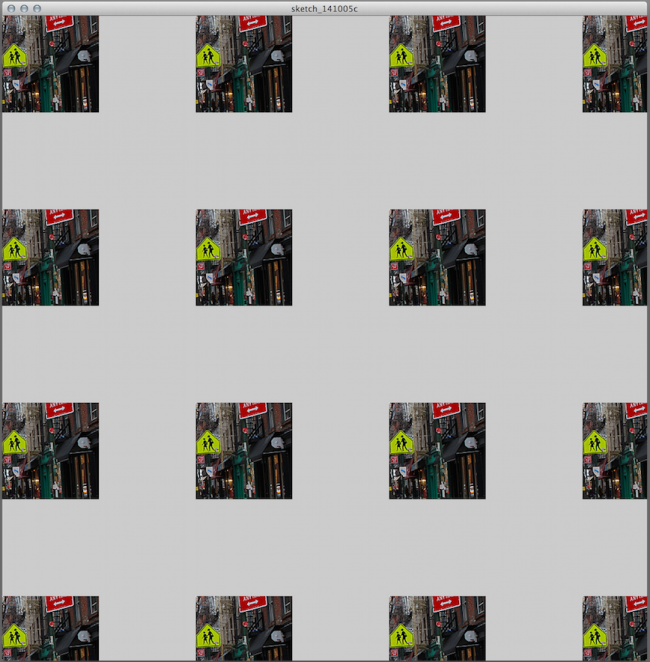#### Howdy, Stranger!

We are about to switch to a new forum software. Until then we have removed the registration on this forum.

# Scaled (mirrored) PImage isn't rendering?

edited October 2014

I am trying to mirror a PImage using pop/push matrix and scale in order to create a pattern, but the only scale that renders is scale(1,1) - the other three don't show up or are transparent or something. Here is what it looks like:There shouldn't be gray there? I have no idea what the issue is and I can't find anyone else who's already posted this problem on the internet, but apologies if the answer is out there and I've been searching by the wrong terms or something. Here is my code, relevant bit is at the bottom:

``````    PImage img;
PImage destination;
FloatList sBlob;

void setup() {
img = loadImage("prince-street-bike-lane.jpg");
destination = createImage(150,150, HSB);
size(1000, 1000);
colorMode(HSB, 360, 100, 100);
sBlob = new FloatList();

img.loadPixels();
float highestAverage = 0;
int highestLocStart = 0;
float blobAverage = 0;
float satch = 0;
int loc = 0;
int sloc = 0;

for (int x = 0; x < img.width - destination.width; x++) {
for (int y = 0; y < img.height - destination.height; y++ ) {
blobAverage = 0;
loc = x + y*img.width;
sBlob.clear();

for (int sx = 0; sx < destination.width; sx++) {
for (int sy = 0; sy < destination.height; sy++ ) {
sloc = loc + sx + sy*img.width;
satch = saturation(img.pixels[sloc]);
sBlob.append(satch);
}
}
for (int i = 0; i < sBlob.size(); i++) {
blobAverage += sBlob.get(i);
}
blobAverage = blobAverage/sBlob.size();
if (blobAverage > highestAverage){
highestAverage = blobAverage;
highestLocStart = loc;
}
print(x + " ");
}
}

for (int x = 0; x < destination.width; x++) {
for (int y = 0; y < destination.height; y++ ) {
int dloc = x + y*destination.width;
int iloc = (x + y*img.width) + highestLocStart;
float h = hue(img.pixels[iloc]);
float s = saturation(img.pixels[iloc]);
float b = brightness(img.pixels[iloc]);
destination.pixels[dloc]  = color(h,s,b);
}
}

destination.updatePixels();

int sx = -1;
int sy = -1;
for (int x = 0; x < width; x += destination.width) {
sx *= -1;
for (int y = 0; y < height; y += destination.height) {
sy *= -1;
pushMatrix();
scale(sx, sy);
image(destination,x,y);
popMatrix();
}
}
}
``````

I'm not super experienced so if you think I'm going about this code in completely the wrong way, feel free to make suggestions. I'm on a mac with current OS and using processing 2.2.1.

Tagged:

## Answers

• 1st off, always place size() as the 1st statement in setup()! 3:-O
http://processing.org/reference/size_.html

2nd, you're asking for 2 things, but your code above got lotsa unrelated things! #-o
You're just making your code harder to debug both for you and for us! :-L
In order to reach a solution, we gotta focus on it, leaving other fancy stuff behind for later! *-:)
For the mirrored request, I've come up w/ a function which returns another PImage as the mirror of its argument:

``````/**
* Mirrored (v1.0)
* by GoToLoop (2014/Oct)
*
* forum.processing.org/two/discussion/7457/
* scaled-mirrored-pimage-isn039t-rendering
*/

PImage getMirror(PImage img) {
PGraphics pg = createGraphics(img.width, img.height, JAVA2D);

pg.beginDraw();
pg.smooth(4);
pg.scale(-1, 1);
pg.image(img, -img.width, 0);
pg.endDraw();

return pg.get();
}

void setup() {
size(800, 600, JAVA2D);
noLoop();
smooth(4);
imageMode(CORNER);
background(0);

set(0, 0, getMirror(loadImage("image.jpg")));
}
``````

For resizing PImage objects, read its resize() method's reference:
http://processing.org/reference/PImage_resize_.html

• hi... I appreciate your answer but I'm not sure it is helpful to me, it complicates the issue. But thank you for your help.

I figured out what the problem was... it was based on a misunderstanding of how the matrix and scale() works. so basically when I was rendering at any scale other than (1,1) such as (-1,1), that image was being drawn outside of the sketch window. I fixed it by replacing the bit of code at the end with the following:

``````          int sx = -1;
int sy = -1;
int mx = 0;
int my = 0;
for (int x = 0; x < width; x += destination.width) {
sx *= -1;
for (int y = 0; y < height; y += destination.height) {
sy *= -1;
if (sx == -1){
mx = x + destination.width;
} else {
mx = x;
}
if (sy == -1){
my = y + destination.height;
} else {
my = y;
}

pushMatrix();
scale(sx, sy);
image(destination,mx*sx,my*sy);
popMatrix();
}
}
``````
• I'm not sure how to mark this as solved. there is no accept button next to my own comment.

Sign In or Register to comment.